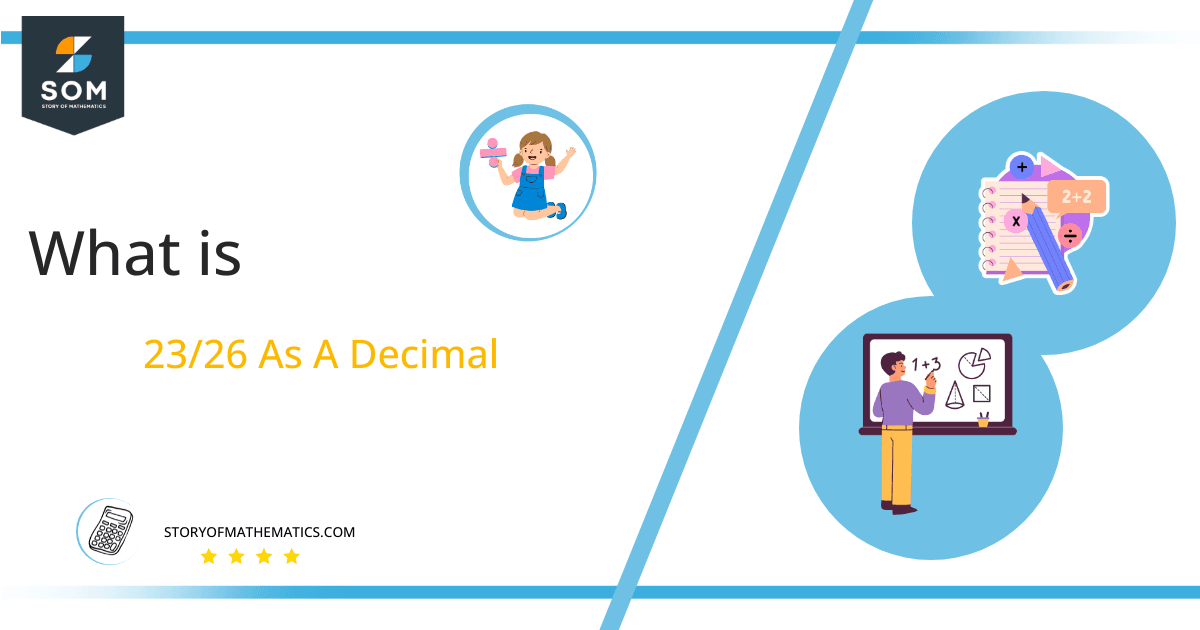# What Is 23/26 as a Decimal + Solution With Free Steps

The fraction 23/26 as a decimal is equal to 0.884.

The fractions can be classified as proper, improper, and mixed fractions. Proper fractions mean those fractions where the numerator is less than the denominator.While in improper fractions, the numerator is greater than the denominator. Mixed fractions have an additional whole number with a fraction.

Here, we are more interested in the division types that result in a Decimal value, as this can be expressed as a Fraction. We see fractions as a way of showing two numbers having the operation of Division between them that result in a value that lies between two Integers.

Now, we introduce the method used to solve said fraction to decimal conversion, called Long Division, which we will discuss in detail moving forward. So, let’s go through the Solution of fraction 23/26.

## Solution

First, we convert the fraction components, i.e., the numerator and the denominator, and transform them into the division constituents, i.e., the Dividend and the Divisor, respectively.

This can be done as follows:

Dividend = 23

Divisor = 26

Now, we introduce the most important quantity in our division process: the Quotient. The value represents the Solution to our division and can be expressed as having the following relationship with the Division constituents:

Quotient = Dividend $\div$ Divisor = 23 $\div$ 26

This is when we go through the Long Division solution to our problem. The following figure shows the long division for the given fraction.Figure 1

## 23/26 Long Division Method

We start solving a problem using the Long Division Method by first taking apart the division’s components and comparing them. As we have 23 and 26, we can see how 23 is Smaller than 26, and to solve this division, we require that 23 be Bigger than 26.

This is done by multiplying the dividend by 10 and checking whether it is bigger than the divisor or not. If so, we calculate the Multiple of the divisor closest to the dividend and subtract it from the Dividend. This produces the Remainder, which we then use as the dividend later.

Now, we begin solving for our dividend 23, which after getting multiplied by 10 becomes 230.

We take this 230 and divide it by 26; this can be done as follows:

230 $\div$ 26 $\approx$ 8

Where:

26 x 8 = 208

This will lead to the generation of a Remainder equal to 230 – 208 = 22. Now this means we have to repeat the process by Converting the 22 into 220 and solving for that:

220 $\div$ 26 $\approx$ 8

Where:

26 x 8 = 208

This, therefore, produces another Remainder equal to 220 – 208 = 12. Now we must solve this problem to Third Decimal Place for accuracy, so we repeat the process with dividend 120.

120 $\div$ 26 $\approx$ 4

Where:

26 x 4 = 104

Finally, we have a Quotient generated after combining the three pieces of it as 0.884, with a Remainder equal to 16.Images/mathematical drawings are created with GeoGebra.